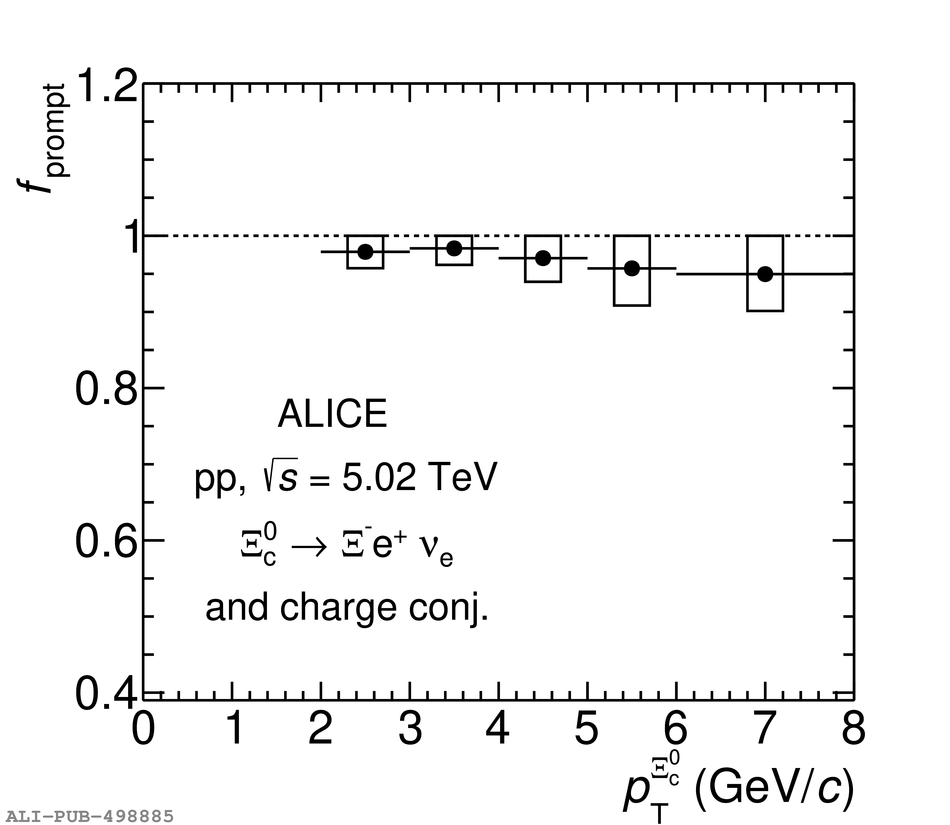# Figure 4

 Left panel: product of acceptance and efficiency for prompt and feed-down $\Xi^0_{\rm c}$ baryons in pp collisions at $\sqrt{s} =$ 5.02 TeV as a function of\pt. Right panel: fraction of prompt $\Xi^0_{\rm c}$ baryons in the raw yield ($f_{\rm prompt}$) as a function of \pt. The systematic uncertainties of $f_{\rm prompt}$ are shown as boxes.